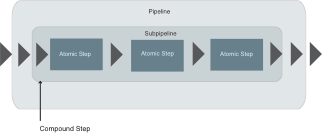XSL-FO - WordML - XSLT

# Compound Steps

Compound Steps contain subpipelines and they allow, amongst other things, nestings. A subpipeline may contain any number of further subpipelines.Figure: example of a Compound Step in XProc

Instead of an Atomic Step as shown in the figure, also a further Compound Step could be displayed, which means a further complete pipeline.

## The important Compound Steps

In the following the important Compound Steps are introduced and each is clarified with the aid of an example.

### p:for-each

``````<p:for-each name? = NCName>
((p:iteration-source? &
(p:output |
p:log)*),
subpipeline)
</p:for-each>``````

The <p:for-each> step is a loop implementation under XProc. In that way documents which are assigned to this step are processed sequentially in a subpipeline. The input has to be provided by a preceding step as a sequence (which means it has to contain several documents). Alternatively, the content could also be provided by <p:iteration-source>.

``````<p:iteration-source select? = XPathExpression>
(p:empty |
(p:pipe |
p:document |
p:inline |
p:data)+)?
</p:iteration-source>``````

<p:iteration-source> is only available in <p:for-each>. As attribute a XPath expression can be used which addresses desired contents from the initial document (from the input port). Alternatively, contents can also be loaded or created via <p:document>, <p:inline> and <p:data>. Then, the imported documents are successively processed in the subpipeline.

#### Example

In the following example the functionality of the <p:for-each> steps is demonstrated.

``````<?xml version="1.0" encoding="UTF-8"?>
<p:declare-step xmlns:p="http://www.w3.org/ns/xproc" xmlns:c="http://www.w3.org/ns/xproc-step" version="1.0">
<p:input port="source">
<p:document href="FilmCollection.xml"/>
</p:input>
<p:output port="result" sequence="true"/>
<p:for-each>
<p:iteration-source select="//Year"/>
<p:output port="result"/>
<p:rename match="/Year" new-name="Date"/>
</p:for-each>
</p:declare-step>``````

The data to be processed is defined by the <p:iteration-source> step. It states that all “Year“ elements of the imported source document shall be processed loopwise. They are sent successively to the <p:rename> step which renames the element as “Date“.

### p:viewport

``````<p:viewport
name? = NCName
match = XSLTMatchPattern>
((p:viewport-source? &
p:output? &
p:log?),
subpipeline)
</p:viewport>``````

By the <p:viewport> Compound Step certain parts of a document can be selected by an appropriate XSLT expression in order to process them in a subpipeline. Alternatively, the desired content can also be provided by <p:viewport-source>.

``````<p:viewport-source>
(p:pipe |
p:document |
p:inline |
p:data)?
</p:viewport-source>``````

<p:viewport-source> is only available as part of <p:viewport>. Here, a document must be indicated. This can be realised by the elements <p:pipe>, <p:document>, <p:inline> or <p:data>. If no document is indicated, a dynamic error will be generated.

#### Example

In the following example only part of the document shall be processed.

``````<?xml version="1.0" encoding="UTF-8"?>
<p:declare-step xmlns:p="http://www.w3.org/ns/xproc" xmlns:c="http://www.w3.org/ns/xproc-step" version="1.0">
<p:input port="source">
<p:document href="FilmCollection.xml"/>
</p:input>
<p:output port="result"/>
<p:viewport match="/FilmCollection/Film/Title">
<p:wrap match="/" wrapper="test"></p:wrap>
</p:viewport>
</p:declare-step>
``````

The XSLT expression in the “match“ attribute of the <p:viewport> step addresses all “Title“ elements from the source document. This part of the document is transfered into the subpipeline of <p:viewport>, in the example only the <p:wrap> step. There, all “Title“ elements are renamed as “test“.

### p:group

``````<p:group name? = NCName>
((p:output |
p:log)*,
subpipeline)
</p:group>``````

<p:group> is a so-called wrapper for an accumulation of several steps. This wrapper compounds these steps and must be applied, for example, in the Multi-Container Step <p:try> within the try area.

#### Example

In the following example a <p:group> step is demonstrated.

``````<?xml version="1.0" encoding="UTF-8"?>
<p:declare-step xmlns:p="http://www.w3.org/ns/xproc" xmlns:c="http://www.w3.org/ns/xproc-step" version="1.0">
<p:input port="source">
<p:empty/>
</p:input>
<p:output port="result"/>
<p:group>
<p:identity>
<p:input port="source">
<p:inline>
<doc>Example</doc>
</p:inline>
</p:input>
</p:identity>
</p:group>
<p:identity/>
</p:declare-step>``````

In this example the <p:identity> step is enclosed with a <p:group> element. The shown source text shall only demonstrate the use of the step.